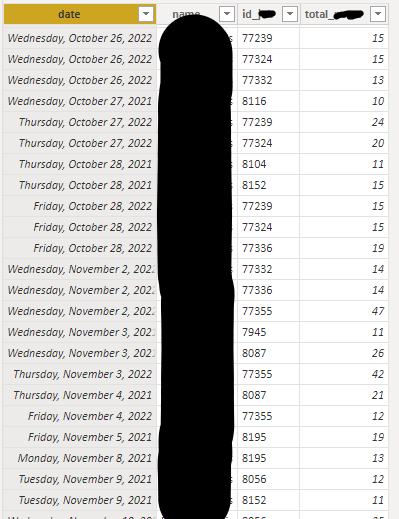cancel
Showing results for
Did you mean:Frequent Visitor

## Values in matrix drill down are not right on a monthly/weekly view

Hello,

I have a table where I have the daily number of actions (column "total") done by each team member (column "name") on each specific task (column "id").Data Table

I use this data in a matrix that serves to recap most of the data in my report with the possibility to drill from year down to month, week and day.Most of my data interacts fine and is summed up accordingly, except the one coming from the table above.

The matrix needs to display the number of active tasks - with the definition of active being that there should have been more than 10 actions done in the period selected.

Therefore, the drill down should be that an action is considered active in the month is there was more than 10 actions in total during the month no matter the day, and 10 in total during the week no matter the day and then 10 in total on a given day.

However, the drill down doesn't work right. The number is right when the granularity is daily but if I drill up to weekly or monthly views, the numbers are wrong.

I have tried various different measures:

active_ =
VAR notes = SUM(daily_[total_])
VAR vac = DISTINCTCOUNT(daily_[id_])
VAR Res = CALCULATE(vac, FILTER(daily_, notes > 10))
Return
Res

active_test = CALCULATE(DISTINCTCOUNT(daily_[id_]), FILTER(daily_, [total_daily] > 10))

active_vacs =
VAR table_ref =
SUMMARIZE(daily_,
daily_[name],
daily_[id_],
daily_[date],
daily_[total_])
VAR dates_inc =
VAR res =
CALCULATE(COUNTROWS(bh_), dates_inc)
RETURN res

And none of them gave the right results. Below, a test I did to check the monthly values, the column 'active_vac' - the one the most at the left - is the correct one (the data is from another table that I can't use for the matrix as it stops at monthly level) and the three other columns are the results given by my different measures tests to get the same monthly values.active_test = CALCULATE(DISTINCTCOUNT(daily_[id_]), FILTER(daily_, [total_daily] > 10))
but its issue was that it didn't sum the different values for each task and only counted the id_ where there was more than 10 notes in a day, while I also want it to sum up by week and month accordingly in the matrix.

Do you know how I could overcome this issue?
Thank you!
2 REPLIES 2Community Support

Hi @Alizee ，

Could you pls share your pbix file ,remember to remove confideential data.

Best Regards

LucienFrequent Visitor

Hello @v-luwang-msft ,

Thank you for your reply, here is my test file for this issue.

https://1drv.ms/u/s!ArUIK7v4HwSbg6NulSN4yd-J-aHtWQ

Thank you very much,
Kind Regards,
Alizée